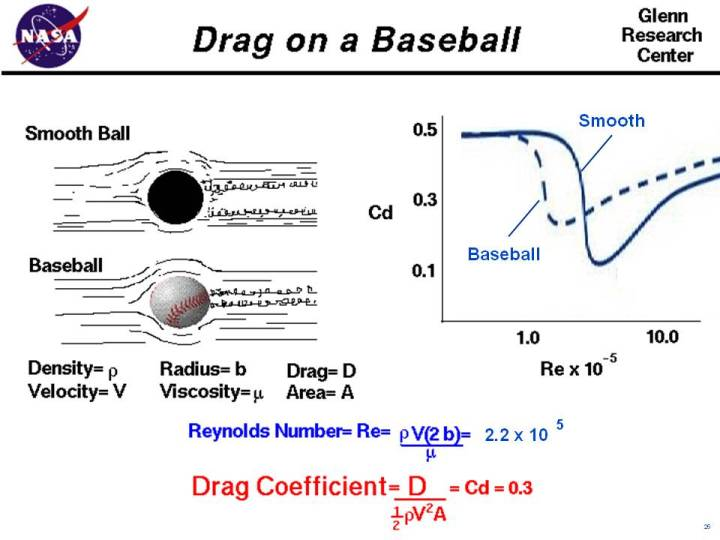+ Text Only Site
+ Non-Flash Version
+ Contact GlennAs an object moves through the air, the air resists the motion of the object. This resistance is called aerodynamic drag. Drag is present on all objects moving through the air from airliners to baseballs. Drag is the component of the aerodynamic force that is aligned and opposite to the flight direction. On this page we will discuss some of the details of the drag on a baseball. The details of how a moving baseball creates drag are fairly complex. If we move with an object through the air, the object appears to stand still and the air moves past the object at the speed of the object. Next to the surface of the object, the molecules of the air stick to the surface, as discussed in the properties of air slide. This thin layer of molecules pulls on the surrounding flow of air. The relative strength of the inertial (momentum) and viscous forces in the flow determines how the flow moves around the object and the value of the drag of the object. The ratio of the inertial force to the viscous force is called the Reynolds number. The equation for the Reynolds number is: Re = rho * V * 2 * b / mu where rho is the air density , V is the velocity, b is the radius of the ball, and mu is the air viscosity. If the value of the Reynolds number is very low (~100), the viscous forces dominate the inertial forces and thick laminar boundary layers are formed on the surface of the object. If the Reynolds number is very large (~ 10^7), the inertial forces dominate the viscous forces and the boundary layer is thin. Unfortunately, the state of the boundary layer is not the only factor that determines the drag on an object. Size , shape , and atmospheric conditions also affect the value of the drag. To predict the value of drag, aerodynamicists use the drag equation: D = .5 * rho * V^2 * A * cd where D is the drag, A is the cross-sectional area, and cd is the drag coefficient a number that represents all of the complex factors affecting drag. Drag coefficients for a specific object are determined experimentally using a model in a wind tunnel. For flow past a ball, determining the drag coefficient gets a little more confusing. The drag on a ball is being generated by the boundary layer separating from the back of the ball. As the flow separates, it creates a viscous wake behind the ball. A large, wide wake generates a large amount of drag; a thin wake produces less drag. The thickness of the wake, and the drag on the ball, depends on the conditions in the boundary layer which, as we have seen, depends on the Reynolds number. For the graph on the right of the figure, we show some experimental data for a smooth ball (in blue). The drag coefficient has a high value at low Re values, then drops down to a lower value from which it continues to increase with increasing Re. The interpretation of the curve is that, at lower Re values, the boundary layer is laminar and the wake is thick. As Re increases, the boundary layer transitions to turbulent, which initially produces a thinner wake, but with increasing speed and Re, the wake thickens and the drag increases. A baseball has a diameter of 2.9 inches (radius = .12 feet). Atmospheric density and viscosity are given on the aerodynamic properties page. For a baseball thrown at 100 mph at sea level on a standard day, the value of the Reynolds number is approximately: Re (baseball) = 2.2 x 10^5 From the curve for a smooth ball, this relatively low Reynolds number value would indicate that the flow in the boundary layer is laminar, and the drag coefficient would be high. For a smooth ball, the drag coefficient is approximately: Cd (smooth) = .5 But we know that a baseball is not a smooth ball. The stitches on the ball produce surface roughness that disturbs the boundary layer, causing the boundary layer to transition at a lower value of the Reynolds number than for a smooth ball. We show a second dashed line on the graph that is more representative of a rough surfaced baseball. From this graph, the value of the drag coefficient for a baseball is approximately: Cd (baseball) = .3 This is the value of drag coefficient that is used in the HitModeler and the CurveBall Expert simulation programs. The figure on this web page was created by Elizabeth Morton, of Magnificat High School, during a "shadowing" experience at NASA Glenn during May of 2007. Activities: Guided Tours Navigation ..NASA Baseball Home Page Beginner's Guide Home Page+ Inspector General Hotline + Equal Employment Opportunity Data Posted Pursuant to the No Fear Act + Budgets, Strategic Plans and Accountability Reports + Freedom of Information Act + The President's Management Agenda + NASA Privacy Statement, Disclaimer, and Accessibility CertificationEditor: Nancy Hall NASA Official: Nancy Hall Last Updated: May 13 2021 + Contact Glenn